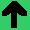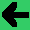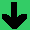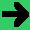# Decision Trees, Symmetric Rules and Transitive Rules

There are three points to this lab. Firstly, you should be able to understand decision trees and implement them in a rule based fashion. Secondly, the concept of symmetry is explored and you should be able to implement rules to handle symmetric functions. Thirdly, the concept of transitivity is explored and you should implement rules to handle transitive functions.

• Decision Trees
• Decision Trees are Trees where each node is a question, each branch is an answer to a question, and each leaf is a result.
• Here is a decision Tree:• Roughly, the idea is that depending of how many of the item (say paper) you have in stock, you make different decisions. If you don't have much, you buy at any cost. If you have a lot you only buy if it is inexpensive.
• First make three rules to handle the root node and its answers.
• Now add rules for the left branch. How does the rules for this branch know they're for it, and not the other branches.
• Now add rules for the central branch.
• Now add rules for the right branch.
• Symmetric and Transitive Rules and Facts
• Symmetry allows you to reorder the operands (arguments).
• For example, addition is symmetric because A+B = B+A
• Subtraction is not symmetric.
• You can make rules to add symmetric facts; for example if (door A B) then (door B A). That is if there is a door from A to B, then there is a door from B to A; most doors behave this way.
• Make a rule for door that adds the symmetric fact.
• Now make up another symmetric rule for a different symmetric function.
• Another mathematical property is transitivity. It involves three operands. If f(X,Y) and f(Y,Z) then f(X,Z). An example of this is less than. If X < Y, and Y < Z then X < Z.
• Make a rule to add transitive facts based on less than.
• Now come up with another transitive function and implement the rule or rules.
• When would you use decision trees?
• When would you use symmetric and transitive functions?